# Review Propositional Logic Chapter 7 1 7 5

• Slides: 26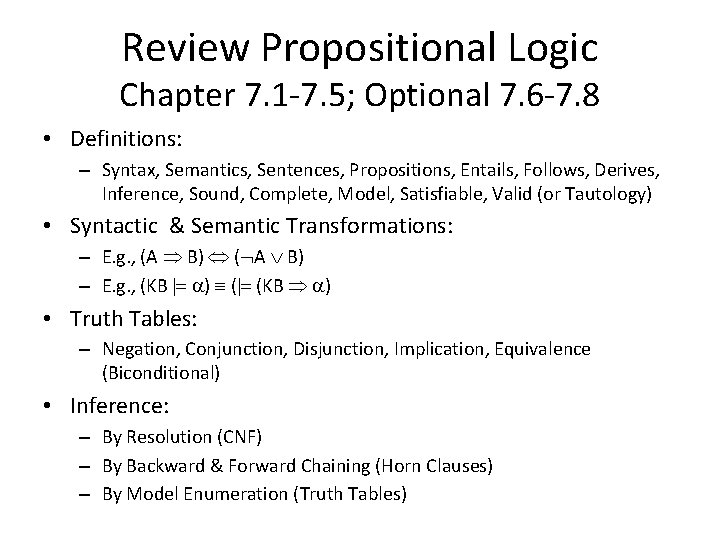Review Propositional Logic Chapter 7. 1 -7. 5; Optional 7. 6 -7. 8 • Definitions: – Syntax, Semantics, Sentences, Propositions, Entails, Follows, Derives, Inference, Sound, Complete, Model, Satisfiable, Valid (or Tautology) • Syntactic & Semantic Transformations: – E. g. , (A B) ( A B) – E. g. , (KB |= ) (|= (KB ) • Truth Tables: – Negation, Conjunction, Disjunction, Implication, Equivalence (Biconditional) • Inference: – By Resolution (CNF) – By Backward & Forward Chaining (Horn Clauses) – By Model Enumeration (Truth Tables)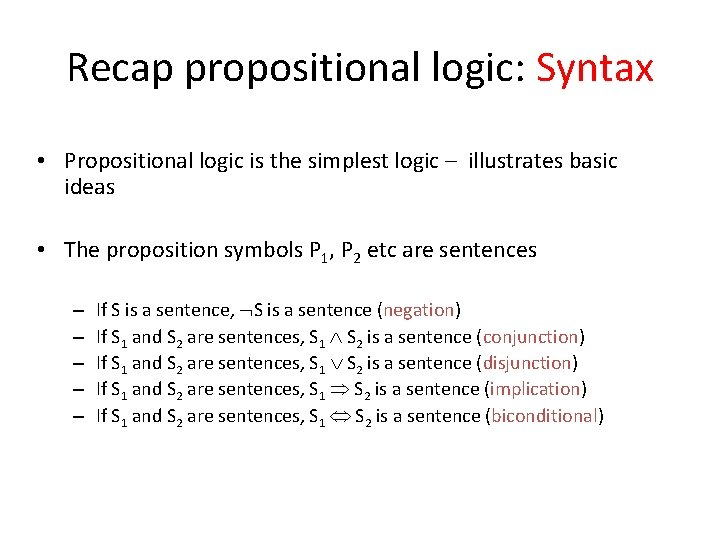Recap propositional logic: Syntax • Propositional logic is the simplest logic – illustrates basic ideas • The proposition symbols P 1, P 2 etc are sentences – – – If S is a sentence, S is a sentence (negation) If S 1 and S 2 are sentences, S 1 S 2 is a sentence (conjunction) If S 1 and S 2 are sentences, S 1 S 2 is a sentence (disjunction) If S 1 and S 2 are sentences, S 1 S 2 is a sentence (implication) If S 1 and S 2 are sentences, S 1 S 2 is a sentence (biconditional)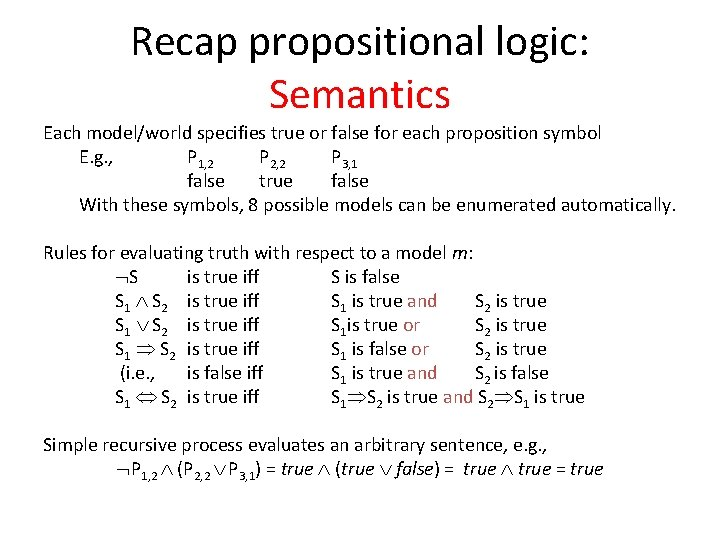Recap propositional logic: Semantics Each model/world specifies true or false for each proposition symbol E. g. , P 1, 2 P 2, 2 P 3, 1 false true false With these symbols, 8 possible models can be enumerated automatically. Rules for evaluating truth with respect to a model m: S is true iff S is false S 1 S 2 is true iff S 1 is true and S 2 is true S 1 S 2 is true iff S 1 is true or S 2 is true S 1 S 2 is true iff S 1 is false or S 2 is true (i. e. , is false iff S 1 is true and S 2 is false S 1 S 2 is true iff S 1 S 2 is true and S 2 S 1 is true Simple recursive process evaluates an arbitrary sentence, e. g. , P 1, 2 (P 2, 2 P 3, 1) = true (true false) = true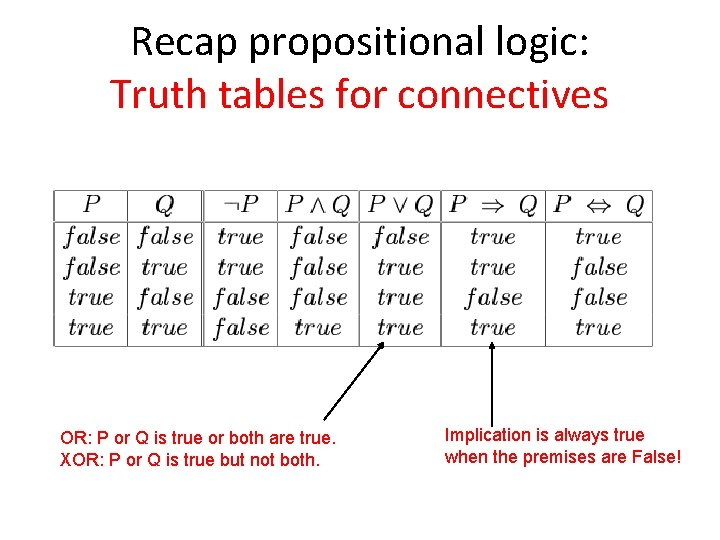Recap propositional logic: Truth tables for connectives OR: P or Q is true or both are true. XOR: P or Q is true but not both. Implication is always true when the premises are False!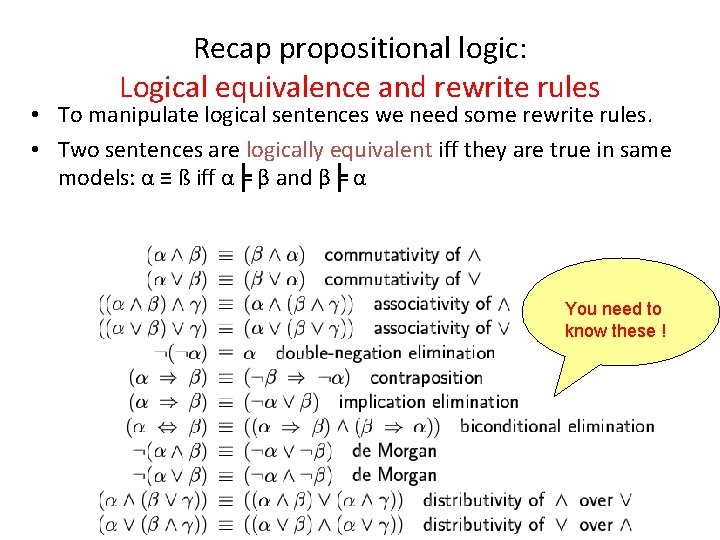Recap propositional logic: Logical equivalence and rewrite rules • To manipulate logical sentences we need some rewrite rules. • Two sentences are logically equivalent iff they are true in same models: α ≡ ß iff α╞ β and β╞ α You need to know these !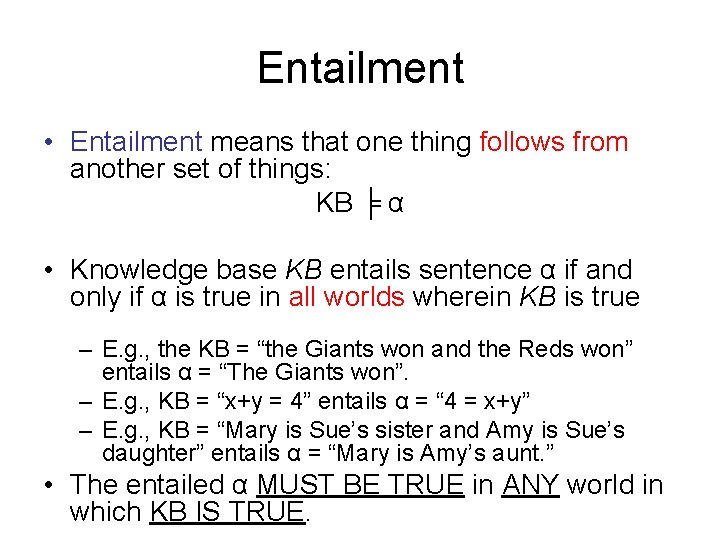Entailment • Entailment means that one thing follows from another set of things: KB ╞ α • Knowledge base KB entails sentence α if and only if α is true in all worlds wherein KB is true – E. g. , the KB = “the Giants won and the Reds won” entails α = “The Giants won”. – E. g. , KB = “x+y = 4” entails α = “ 4 = x+y” – E. g. , KB = “Mary is Sue’s sister and Amy is Sue’s daughter” entails α = “Mary is Amy’s aunt. ” • The entailed α MUST BE TRUE in ANY world in which KB IS TRUE.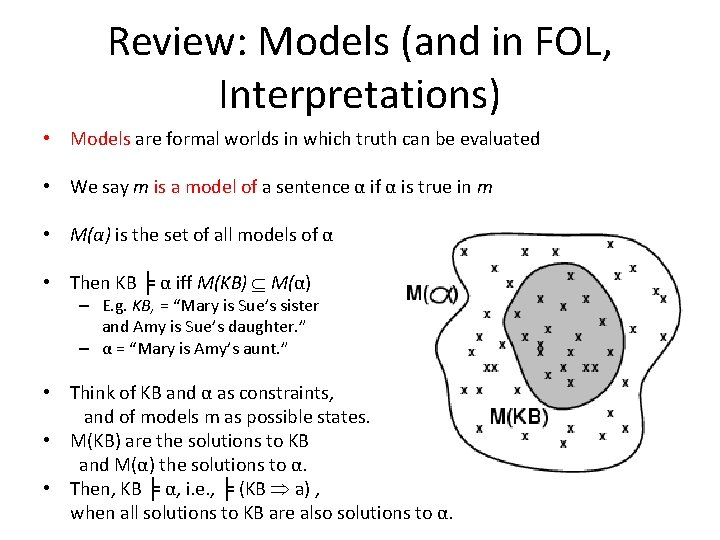Review: Models (and in FOL, Interpretations) • Models are formal worlds in which truth can be evaluated • We say m is a model of a sentence α if α is true in m • M(α) is the set of all models of α • Then KB ╞ α iff M(KB) M(α) – E. g. KB, = “Mary is Sue’s sister and Amy is Sue’s daughter. ” – α = “Mary is Amy’s aunt. ” • Think of KB and α as constraints, and of models m as possible states. • M(KB) are the solutions to KB and M(α) the solutions to α. • Then, KB ╞ α, i. e. , ╞ (KB a) , when all solutions to KB are also solutions to α.Wumpus models All possible models in this reduced Wumpus world. What can we infer?Review: Wumpus models • KB = all possible wumpus-worlds consistent with the observations and the “physics” of the Wumpus world.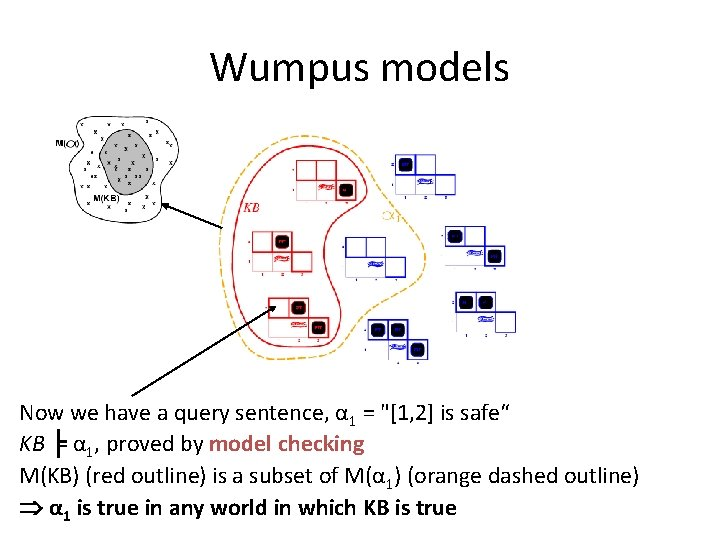Wumpus models Now we have a query sentence, α 1 = "[1, 2] is safe“ KB ╞ α 1, proved by model checking M(KB) (red outline) is a subset of M(α 1) (orange dashed outline) α 1 is true in any world in which KB is true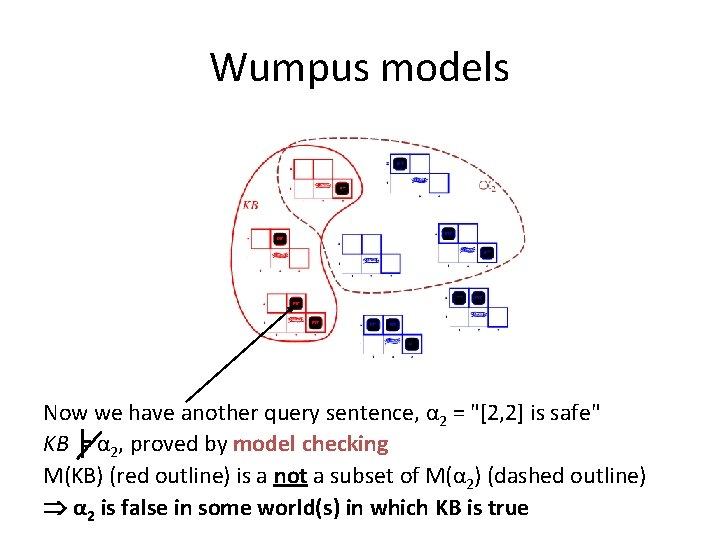Wumpus models Now we have another query sentence, α 2 = "[2, 2] is safe" KB ╞ α 2, proved by model checking M(KB) (red outline) is a not a subset of M(α 2) (dashed outline) α 2 is false in some world(s) in which KB is trueRecap propositional logic: Validity and satisfiability A sentence is valid if it is true in all models, e. g. , True, A A, (A B)) B Validity is connected to inference via the Deduction Theorem: KB ╞ α if and only if (KB α) is valid A sentence is satisfiable if it is true in some model e. g. , A B, C A sentence is unsatisfiable if it is false in all models e. g. , A A Satisfiability is connected to inference via the following: KB ╞ A if and only if (KB A) is unsatisfiable (there is no model for which KB is true and A is false)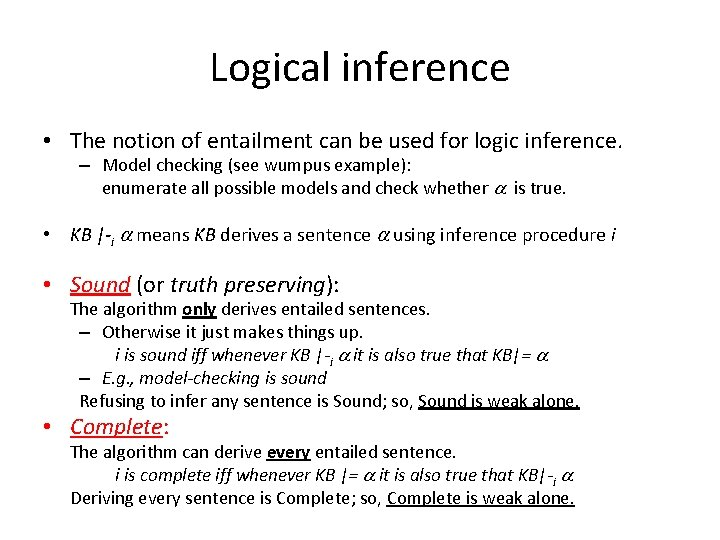Logical inference • The notion of entailment can be used for logic inference. – Model checking (see wumpus example): enumerate all possible models and check whether is true. • KB |-i means KB derives a sentence using inference procedure i • Sound (or truth preserving): The algorithm only derives entailed sentences. – Otherwise it just makes things up. i is sound iff whenever KB |-i it is also true that KB|= – E. g. , model-checking is sound Refusing to infer any sentence is Sound; so, Sound is weak alone. • Complete: The algorithm can derive every entailed sentence. i is complete iff whenever KB |= it is also true that KB|-i Deriving every sentence is Complete; so, Complete is weak alone.Inference by Resolution • KB is represented in CNF – KB = AND of all the sentences in KB – KB sentence = clause = OR of literals – Literal = propositional symbol or its negation • Find two clauses in KB, one of which contains a literal and the other its negation – – Cancel the literal and its negation Bundle everything else into a new clause Add the new clause to KB Repeat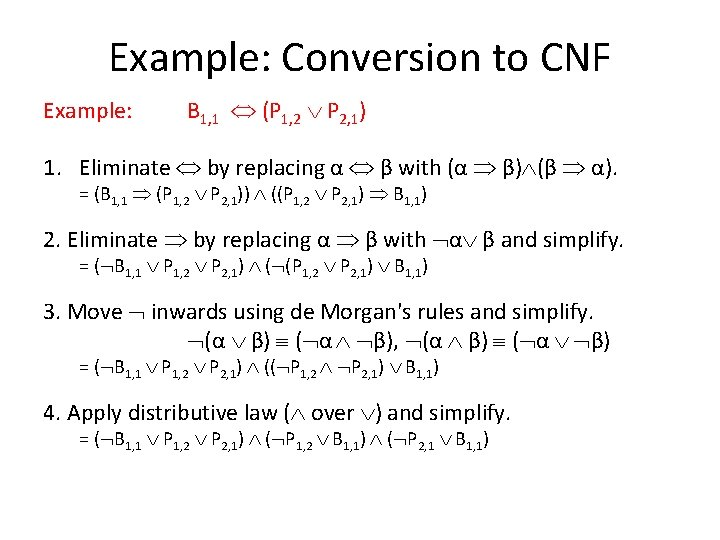Example: Conversion to CNF Example: B 1, 1 (P 1, 2 P 2, 1) 1. Eliminate by replacing α β with (α β) (β α). = (B 1, 1 (P 1, 2 P 2, 1)) ((P 1, 2 P 2, 1) B 1, 1) 2. Eliminate by replacing α β with α β and simplify. = ( B 1, 1 P 1, 2 P 2, 1) ( (P 1, 2 P 2, 1) B 1, 1) 3. Move inwards using de Morgan's rules and simplify. (α β) ( α β), (α β) ( α β) = ( B 1, 1 P 1, 2 P 2, 1) (( P 1, 2 P 2, 1) B 1, 1) 4. Apply distributive law ( over ) and simplify. = ( B 1, 1 P 1, 2 P 2, 1) ( P 1, 2 B 1, 1) ( P 2, 1 B 1, 1)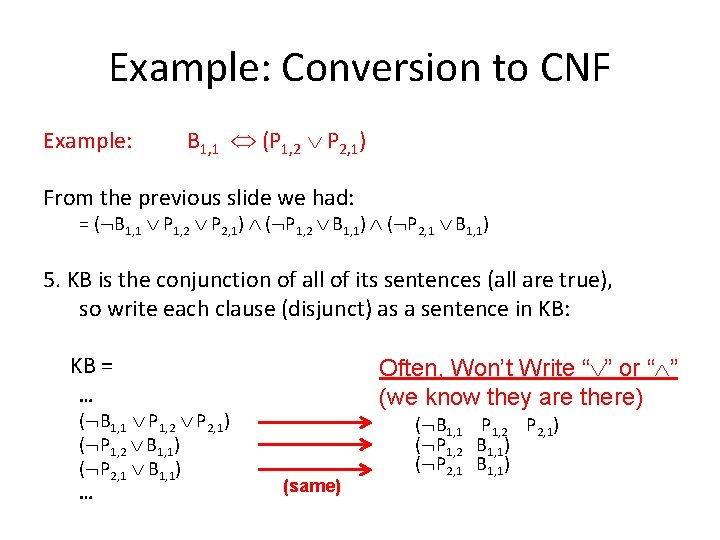Example: Conversion to CNF Example: B 1, 1 (P 1, 2 P 2, 1) From the previous slide we had: = ( B 1, 1 P 1, 2 P 2, 1) ( P 1, 2 B 1, 1) ( P 2, 1 B 1, 1) 5. KB is the conjunction of all of its sentences (all are true), so write each clause (disjunct) as a sentence in KB: KB = … ( B 1, 1 P 1, 2 P 2, 1) ( P 1, 2 B 1, 1) ( P 2, 1 B 1, 1) … Often, Won’t Write “ ” or “ ” (we know they are there) (same) ( B 1, 1 P 1, 2 P 2, 1) ( P 1, 2 B 1, 1) ( P 2, 1 B 1, 1)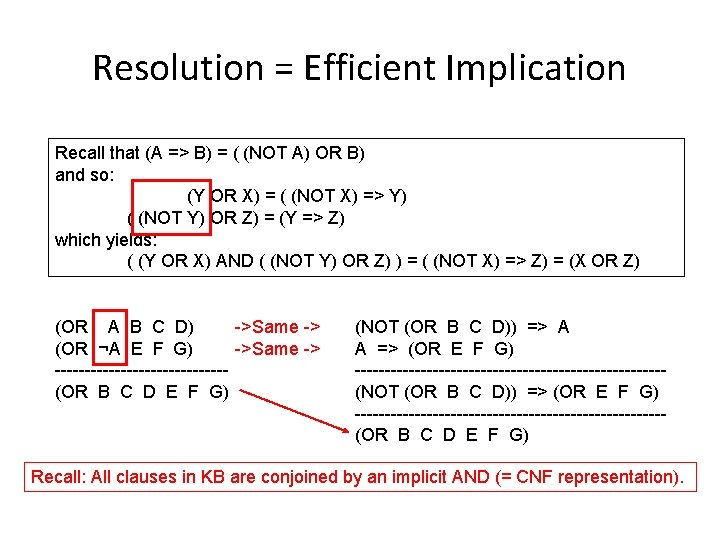Resolution = Efficient Implication Recall that (A => B) = ( (NOT A) OR B) and so: (Y OR X) = ( (NOT X) => Y) ( (NOT Y) OR Z) = (Y => Z) which yields: ( (Y OR X) AND ( (NOT Y) OR Z) ) = ( (NOT X) => Z) = (X OR Z) ->Same -> (OR A B C D) ->Same -> (OR ¬A E F G) --------------(OR B C D E F G) (NOT (OR B C D)) => A A => (OR E F G) --------------------------(NOT (OR B C D)) => (OR E F G) --------------------------(OR B C D E F G) Recall: All clauses in KB are conjoined by an implicit AND (= CNF representation).Resolution Examples • Resolution: inference rule for CNF: sound and complete! * “If A or B or C is true, but not A, then B or C must be true. ” “If A is false then B or C must be true, or if A is true then D or E must be true, hence since A is either true or false, B or C or D or E must be true. ” “If A or B is true, and not A or B is true, then B must be true. ” Simplification is done always. * Resolution is “refutation complete” in that it can prove the truth of any entailed sentence by refutation.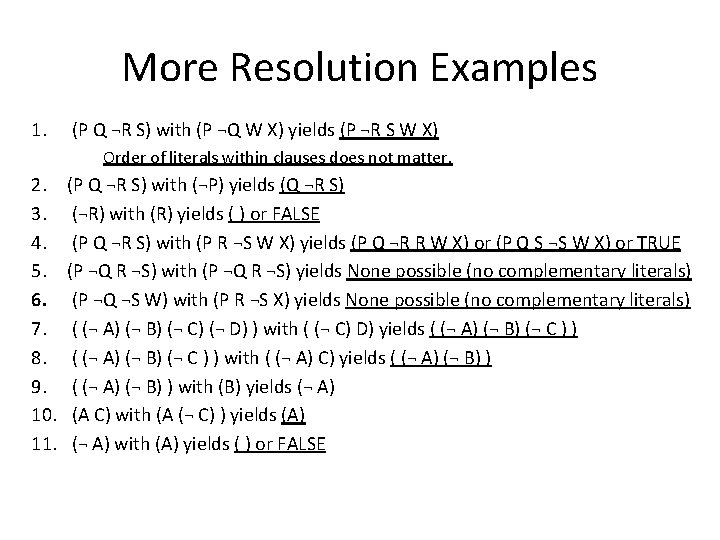More Resolution Examples 1. (P Q ¬R S) with (P ¬Q W X) yields (P ¬R S W X) Order of literals within clauses does not matter. 2. 3. 4. 5. 6. 7. 8. 9. 10. 11. (P Q ¬R S) with (¬P) yields (Q ¬R S) (¬R) with (R) yields ( ) or FALSE (P Q ¬R S) with (P R ¬S W X) yields (P Q ¬R R W X) or (P Q S ¬S W X) or TRUE (P ¬Q R ¬S) with (P ¬Q R ¬S) yields None possible (no complementary literals) (P ¬Q ¬S W) with (P R ¬S X) yields None possible (no complementary literals) ( (¬ A) (¬ B) (¬ C) (¬ D) ) with ( (¬ C) D) yields ( (¬ A) (¬ B) (¬ C ) ) with ( (¬ A) C) yields ( (¬ A) (¬ B) ) with (B) yields (¬ A) (A C) with (A (¬ C) ) yields (A) (¬ A) with (A) yields ( ) or FALSE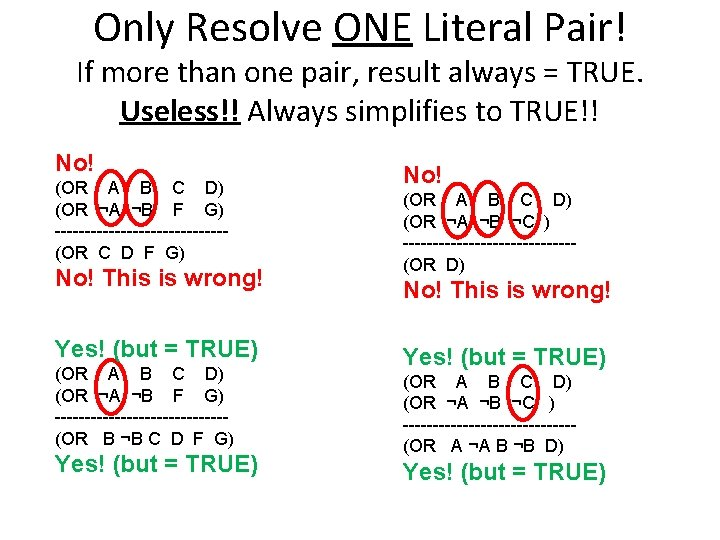Only Resolve ONE Literal Pair! If more than one pair, result always = TRUE. Useless!! Always simplifies to TRUE!! No! (OR A B C D) (OR ¬A ¬B F G) --------------(OR C D F G) No! This is wrong! Yes! (but = TRUE) (OR A B C D) (OR ¬A ¬B F G) --------------(OR B ¬B C D F G) Yes! (but = TRUE) No! (OR A B C D) (OR ¬A ¬B ¬C ) --------------(OR D) No! This is wrong! Yes! (but = TRUE) (OR A B C D) (OR ¬A ¬B ¬C ) --------------(OR A ¬A B ¬B D) Yes! (but = TRUE)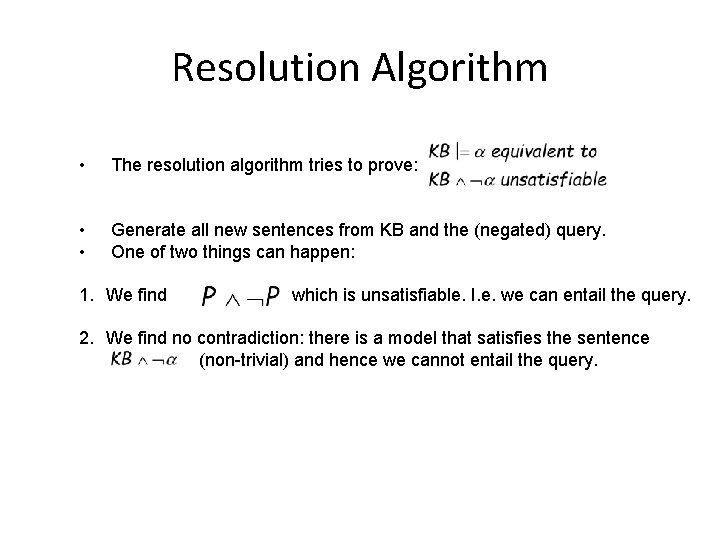Resolution Algorithm • The resolution algorithm tries to prove: • • Generate all new sentences from KB and the (negated) query. One of two things can happen: 1. We find which is unsatisfiable. I. e. we can entail the query. 2. We find no contradiction: there is a model that satisfies the sentence (non-trivial) and hence we cannot entail the query.Resolution example Resulting Knowledge Base stated in CNF • “Laws of Physics” in the Wumpus World: ( B 1, 1 P 1, 2 P 2, 1) ( P 1, 2 B 1, 1) ( P 2, 1 B 1, 1) • Particular facts about a specific instance: ( B 1, 1) • Negated goal or query sentence: (P 1, 2)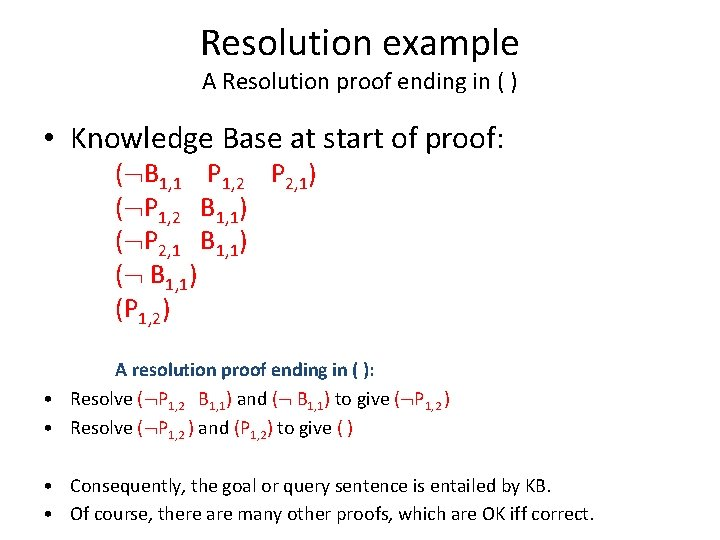Resolution example A Resolution proof ending in ( ) • Knowledge Base at start of proof: ( B 1, 1 P 1, 2 P 2, 1) ( P 1, 2 B 1, 1) ( P 2, 1 B 1, 1) (P 1, 2) A resolution proof ending in ( ): • Resolve ( P 1, 2 B 1, 1) and ( B 1, 1) to give ( P 1, 2 ) • Resolve ( P 1, 2 ) and (P 1, 2) to give ( ) • Consequently, the goal or query sentence is entailed by KB. • Of course, there are many other proofs, which are OK iff correct.Detailed Resolution Proof Example • In words: If the unicorn is mythical, then it is immortal, but if it is not mythical, then it is a mortal mammal. If the unicorn is either immortal or a mammal, then it is horned. The unicorn is magical if it is horned. Prove that the unicorn is both magical and horned. ( (NOT Y) (NOT R) ) (H R) • • • (M Y) ( (NOT H) G) (R Y) ( (NOT G) (NOT H) ) (H (NOT M) ) Fourth, produce a resolution proof ending in ( ): Resolve (¬H ¬G) and (¬H G) to give (¬H) Resolve (¬Y ¬R) and (Y M) to give (¬R M) Resolve (¬R M) and (R H) to give (M H) Resolve (M H) and (¬M H) to give (H) Resolve (¬H) and (H) to give ( ) • Of course, there are many other proofs, which are OK iff correct.Horn Clauses • Resolution can be exponential in space and time. • If we can reduce all clauses to “Horn clauses” inference is linear in space and time A clause with at most 1 positive literal. e. g. • Every Horn clause can be rewritten as an implication with a conjunction of positive literals in the premises and at most a single positive literal as a conclusion. e. g. • 1 positive literal and 1 negative literal: definite clause (e. g. , above) • 0 positive literals: integrity constraint or goal clause e. g. states that (A B) must be false • 0 negative literals: fact e. g. , (A) (True A) states that A must be true. • Forward Chaining and Backward chaining are sound and complete with Horn clauses and run linear in space and time.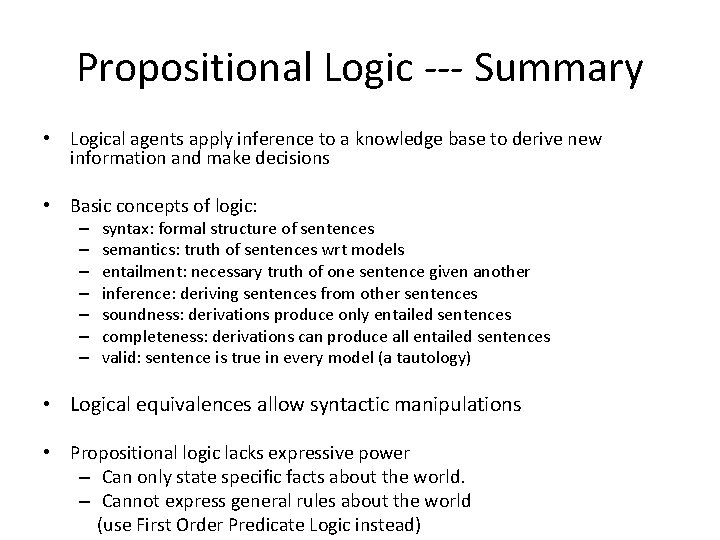Propositional Logic --- Summary • Logical agents apply inference to a knowledge base to derive new information and make decisions • Basic concepts of logic: – – – – syntax: formal structure of sentences semantics: truth of sentences wrt models entailment: necessary truth of one sentence given another inference: deriving sentences from other sentences soundness: derivations produce only entailed sentences completeness: derivations can produce all entailed sentences valid: sentence is true in every model (a tautology) • Logical equivalences allow syntactic manipulations • Propositional logic lacks expressive power – Can only state specific facts about the world. – Cannot express general rules about the world (use First Order Predicate Logic instead)